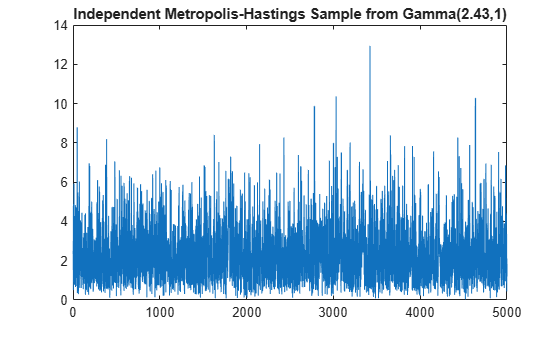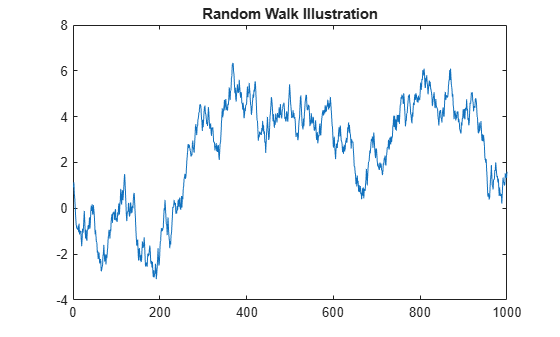# mhsample

Metropolis-Hastings sample

## Syntax

```smpl = mhsample(start,nsamples,'pdf',pdf,'proppdf',proppdf, 'proprnd',proprnd) smpl = mhsample(...,'symmetric',sym) smpl = mhsample(...,'burnin',K) smpl = mhsample(...,'thin',m) smpl = mhsample(...,'nchain',n) [smpl,accept] = mhsample(...) ```

## Description

```smpl = mhsample(start,nsamples,'pdf',pdf,'proppdf',proppdf, 'proprnd',proprnd)``` draws `nsamples` random samples from a target stationary distribution `pdf` using the Metropolis-Hastings algorithm.

`start` is a row vector containing the start value of the Markov Chain, `nsamples` is an integer specifying the number of samples to be generated, and `pdf`, `proppdf`, and `proprnd` are function handles created using `@`. `proppdf` defines the proposal distribution density, and `proprnd` defines the random number generator for the proposal distribution. `pdf` and `proprnd` take one argument as an input with the same type and size as `start`. `proppdf` takes two arguments as inputs with the same type and size as `start`.

`smpl` is a column vector or matrix containing the samples. If the log density function is preferred, `'pdf'` and `'proppdf'` can be replaced with `'logpdf'` and `'logproppdf'`. The density functions used in Metropolis-Hastings algorithm are not necessarily normalized.

The proposal distribution q(x,y) gives the probability density for choosing x as the next point when y is the current point. It is sometimes written as q(x|y).

If the `proppdf` or `logproppdf` satisfies q(x,y) = q(y,x), that is, the proposal distribution is symmetric, `mhsample` implements Random Walk Metropolis-Hastings sampling. If the `proppdf` or `logproppdf` satisfies q(x,y) = q(x), that is, the proposal distribution is independent of current values, `mhsample` implements Independent Metropolis-Hastings sampling.

`smpl = mhsample(...,'symmetric',sym)` draws `nsamples` random samples from a target stationary distribution `pdf` using the Metropolis-Hastings algorithm. `sym` is a logical value that indicates whether the proposal distribution is symmetric. The default value is false, which corresponds to the asymmetric proposal distribution. If `sym` is true, for example, the proposal distribution is symmetric, `proppdf` and `logproppdf` are optional.

`smpl = mhsample(...,'burnin',K)` generates a Markov chain with values between the starting point and the `k`th point omitted in the generated sequence. Values beyond the `k`th point are kept. `k` is a nonnegative integer with default value of `0`.

`smpl = mhsample(...,'thin',m)` generates a Markov chain with `m-1` out of `m` values omitted in the generated sequence. `m` is a positive integer with default value of `1`.

`smpl = mhsample(...,'nchain',n)` generates `n` Markov chains using the Metropolis-Hastings algorithm. `n` is a positive integer with a default value of 1. `smpl` is a matrix containing the samples. The last dimension contains the indices for individual chains.

`[smpl,accept] = mhsample(...)` also returns `accept`, the acceptance rate of the proposed distribution. `accept` is a scalar if a single chain is generated and is a vector if multiple chains are generated.

## Examples

collapse all

Use Independent Metropolis-Hastings sampling to estimate the second order moment of a Gamma distribution.

```rng default; % For reproducibility alpha = 2.43; beta = 1; pdf = @(x)gampdf(x,alpha,beta); % Target distribution proppdf = @(x,y)gampdf(x,floor(alpha),floor(alpha)/alpha); proprnd = @(x)sum(... exprnd(floor(alpha)/alpha,floor(alpha),1)); nsamples = 5000; smpl = mhsample(1,nsamples,'pdf',pdf,'proprnd',proprnd,... 'proppdf',proppdf);```

Plot the results.

```xxhat = cumsum(smpl.^2)./(1:nsamples)'; figure; plot(1:nsamples,xxhat)```Use Random Walk Metropolis-Hastings sampling to generate sample data from a standard normal distribution.

```rng default % For reproducibility delta = .5; pdf = @(x) normpdf(x); proppdf = @(x,y) unifpdf(y-x,-delta,delta); proprnd = @(x) x + rand*2*delta - delta; nsamples = 15000; x = mhsample(1,nsamples,'pdf',pdf,'proprnd',proprnd,'symmetric',1);```

Plot the sample data.

```figure; h = histfit(x,50); h(1).FaceColor = [.8 .8 1];```## Version History

Introduced in R2006a